# I.E. Irodov Solutions on Elastic Waves

I.E. Irodov Solutions on Chapter 4.3. Elastic Waves (Acoustics) are available on this page. I.E. Irodov Solutions Chapter 4.3 Elastic Waves consists of answers to all the major questions which are found in the I.E. Irodov book and those questions that are frequent in competitive exams. Questions are based on topics like wave motion, wave pulse on a string, phase difference, wave oscillation, transverse and longitudinal waves. In order to help students understand the concepts clearly as well as learn the right answers, we are offering a comprehensive set of I.E. Irodov Solutions for Physics. The solutions will help students understand the topics of elastic waves more clearly.

### I.E. Irodov Solutions on Elastic Waves

1: How long will it take sound waves to travel the distance l between the points A and B if the air temperature between them varies linearly from T1 to T2? The velocity of sound propagation in air is equal to v = α√T, where a is a constant.

Solution:

1. Since the temperature varies linearly we can write the temperature as a function of x, which is, the distance from the point A towards B. i.e.,

T = T1 + (T2-T1)x/l [o < x < l]

Hence, dT = (T2-T1)/l dx ……. (1)

In order to travel an elemental distance of dx which is at a distance of x from A it will take a time.

dt = dx/α√T ….(2)

From (1) and (2), we get

$dt =\frac{l}{\alpha \sqrt{T}}(\frac{ldT}{T_2-T_1})$

On integrating, we get

$\int_0^t dt = \frac{l}{\alpha(T_2-T_1)} \int_{T_1}^{T_2} \frac{dT}{\sqrt{T}}$

Or t = [2l/(T2-T1)] (√T2-√T1)

Hence the sought time is t = 2l/α (√T2+√T1)

2: A plane harmonic wave with frequency ω propagates at a velocity v in a direction forming angles α, β, γ with the x, y, z axes. Find the phase difference between the oscillations at the points of medium with coordinates x1, y1, z1 and x2, y2, z2.

Solution:

1.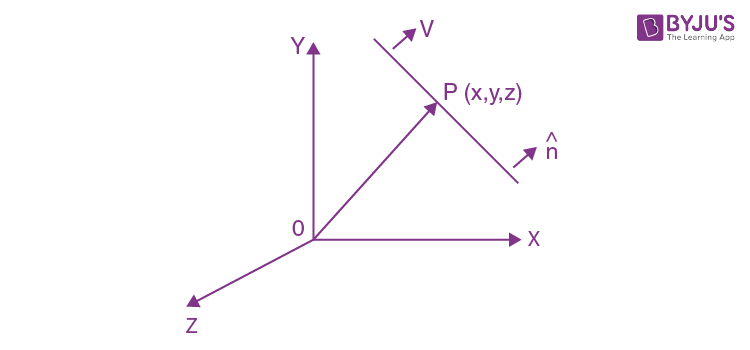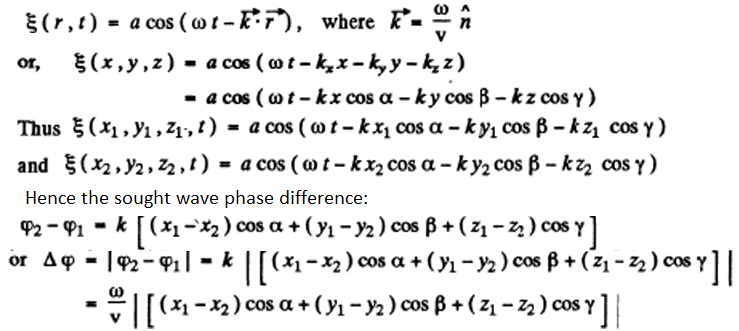Here, vector k is called the wave vector and n is the unit vector normal to the wave surface in the direction of the propagation of wave.

3: A plane wave of frequency ω propagates so that a certain phase of oscillation moves along the x, y, z axes with velocities v1, v2, v3 respectively. Find the wave vector k, assuming the unit vectors ex, ey, ez of the coordinate axes to be assigned.

Solution:

1. The phase of the oscillation can be written as

ϕ =ωt – kr

where k and r are two vectors.

When the wave moves along the x-axis

ϕ =ωt – kxr (here ky = kz = 0)

Since the velocity associated with this wave is v1

we have kx = ω/v1

Similarly, ky = ω/v2 and kz = ω/v3

$\vec{k} = \frac{\omega}{v_1}\hat{e_x}+\frac{\omega}{v_2}\hat{e_y}+\frac{\omega}{v_3}\hat{e_z}$

4: A plane elastic wave $\xi=acos(\omega t-kx)$ propagates in a medium K. Find the equation of this wave in a reference frame K' moving in the positive direction of the x axis with a constant velocity V relative to the medium K. Investigate the expression obtained.

Solution:

1. The wave equation propagating in the direction of +ve x axis in medium K is give as

$\xi=acos(\omega t-kx)$

so, $\xi=acosk(v t-x)$

where k = ω/v and v is the wave velocity.

In the reference frame K’, the wave velocity will be (v - V) propagating in the direction of +ve x-axis and x will be x'. Thus, the sought wave equation.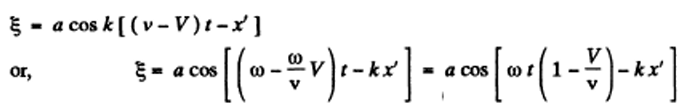5: Demonstrate that any differentiable function f(t + ax), where a is a constant, provides a solution of wave equation. What is the physical meaning of the constant α?

Solution:

1. This follows on actually putting

$\xi = f(t+\alpha x)$

In the wave equation :

$\frac{\partial^2 \xi}{\partial x^2}=\frac{1}{v^2}\frac{\partial^2 \xi}{\partial t^2}$

Then, (1/v2) f’’(t+αx) = α2f’’(t+αx)

So the wave equation is satisfied if

α =1/v’ and α = -1/v’

That is the physical meaning of the constant α.

6: The equation of a travelling plane sound wave has the form ξ = 60 cos (1800t - 5.3x), where ξ is expressed in micrometres, t in seconds, and x in metres. Find:

(a) the ratio of the displacement amplitude, with which the particles of medium oscillate, to the wavelength;

(b) the velocity oscillation amplitude of particles of the medium and its ratio to the wave propagation velocity;

(c) the oscillation amplitude of relative deformation of the medium and its relation to the velocity oscillation amplitude of particles of the medium.

Solution:

1. The given wave equation

ξ = 60 cos(1800t - 5.3x)

= a cos(ωt - kx), where a = 60 x 10-6 m

ω =1800 per sec and k = 5.3 per metre

As, k = 2π/λ so λ = 2π/k

Also, k = ω/v, so, v = ω/k = 340 m/s

(a)Sough ratio = a/ λ = ak/2 π = 5.1 x 10-5

(b) ξ = a cos(ω t - kx)

Partial derivative of ξ with time is -aωsin(ωt - kx)

So velocity oscillation amplitude

vm = aω = 0.11 m/s ….(1)

And the sought ratio of velocity oscillation amplitude to the wave propagation velocity = vm/v = 0.11/340 = 3.2 x 10-4

(c) Relative deformation = ak sin(ωt – kx)

So, relative deformation amplitude = ak = (60x10-6x5.3)m = 3.2 x 10-4 m. …(2)

From (1) and (2)

$(\frac{\partial \xi}{\partial x})_m=ak=\frac{a \omega}{v}=(1/v)(\frac{\partial \xi}{\partial t})_m$

Where v = 340 m/s is the wave velocity.

7: A plane wave ξ = a cos (ωt-kx) propagates in a homogeneous elastic medium. For the moment t = 0 draw

(a) the plots of ξ, $\frac{\partial \xi}{\partial t}\ and\ \frac{\partial \xi}{\partial x}$

(b) the velocity direction of the particles of the medium at the points where ξ = 0, for the cases of longitudinal and transverse waves;

(c) the approximate plot of density distribution ρ(x) of the medium for the case of longitudinal waves.

Solution:

1. (a) The given equation is,

ξ = a cos (ωt-kx)

At t = 0

Ξ = a cos kx

Now, d ξ/dt = -a ω sin(ωt – kx)

d ξ/dt = a ω sin(kx) at t =0

also, d ξ/dx = a ω sin(ωt – kx)

at t = o

d ξ/dx = -a kω sin(kx)

Hence all the graphs are similar having different amplitudes.

(b) At the points, where ξ= 0, the velocity direction is positive, i.e., along + ve x-axis in the case of longitudinal and + ve y- axis in the case of transverse waves, where dξ/dt is positive and vice versa.

8: A plane elastic wave ξ= ae-Yx cos (ωt -kx), where a, γ, ω, and k are constants, propagates in a homogeneous medium. Find the phase difference between the oscillations at the points where the particles' displacement amplitudes differ by η = 1.0%, if γ = = 0.42 m-1 and the wavelength is γ = 50 cm.

Solution:

1. In the given wave equation the particle’s displacement amplitude = ae-yx

Let two points x1 and x2 , between which the displacement amplitude differ by η = 1 %

So, ae-γx1 - ae-γx2 = η ae-γx1

e-γx1(1 – η) = e-γx2

ln(1- η) – γx1 = – γx2

x2-x1 = - ln(1- η)/ γ

The path difference, 2 πx/λ

path difference= (-2 π/λ)( ln(1- η)/ γ) = 0.3 rad

9: Find the radius vector defining the position of a point source of spherical waves if that source is known to be located on the straight line between the points with radius vectors r1 and r2 at which the oscillation amplitudes of particles of the medium are equal to a1 and a2. The damping of the wave is negligible, the medium is homogeneous.

Solution:

1. Let S be the source whose position vector relative to the reference point O is vector r. Since intensities are inversely proportional to the square of distances,

[intensity at P(I1)]/ [intensity at Q(I2)] = d22/ d21

Where d1 = PS and d2 = QS

But intensity is proportional to the square of amplitude

a22/ a21 = d22/ d21

or a1d1 = a2d2 = k(say)

Thus d1 = k/a1 and d2 = k/a2

Let n be the unit vector along PQ directed from P to Q.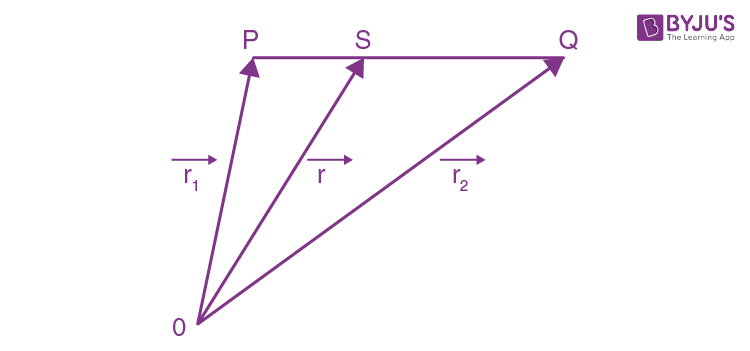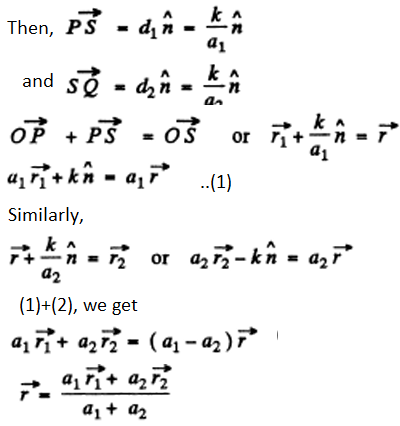10: A point isotropic source generates sound oscillations with frequency v = 1.45 kHz. At a distance r = 5.0 m from the source the displacement amplitude of particles of the medium is equal to a0 = 50 pm, and at the point A located at a distance r = 10.0 m from the source the displacement amplitude is η = 3.0 times less than a0. Find:

(a) the damping coefficient γ of the wave;

(b) the velocity oscillation amplitude of particles of the medium at the point A.

Solution:

1. (a) We know that the equation of a spherical wave in a homogeneous absorbing medium of wave damping coefficient γ is:

ξ = [a0'e-γr/r] cos(ωt - kr)

Particle’s displacement amplitude equals, a0'e-γr/r

According to the given conditions, r = ro, ao = a0'e-γr/ro …(1)

When r = ro, a0/ η = a0'e-γr/r …(2)

Form (1) and (2)

eγ(r- ro) = η (r0/r)

γ(r- ro)=ln(ηro) – ln r

or, γ = 0.08 m-1.

(b)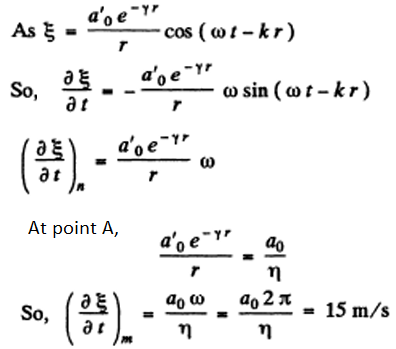11: Two plane waves propagate in a homogeneous elastic medium, one along the x axis and the other along the y axis: ξ1 = a cos (ωt -kx), ξ2 = a cos (ωt -ky). Find the wave motion pattern of particles in the plane xy if both waves

(a) are transverse and their oscillation directions coincide;

(b) are longitudinal.

Solution:

1. (a) Equation of the resultant wave,

ξ= ξ1 + ξ2 = 2a cos k[(y-x)/2] cos[ωt - k[(y+x)/2]]

=a’ cos[ωt - k[(y+x)/2]] where a’ = 2a cos k[(y-x)/2]

Now, the equation of wave pattern is, x + y = k , (a Const.)

For antinodes, i.e. maximum intensity

cos k[(y-x)/2] = cos nπ

-(x-y) = 2nπ/k and (x-y) = 2nπ/k = nλ

y = x + nλ and y = x – nλ where n = 0,1,2,3,….

Hence, the particles of the medium at the points, lying on the solid straight lines , oscillate with maximum amplitude.

For nodes, i.e. minimum intensity,

Cos[k(y-x)/2] = 0

Or y = x + (2n+1) (λ/2) and y = x - (2n+1) (λ/2)

Hence the particles at the points, lying on dotted lines do not oscillate.

(b) When the waves are longitudinal,

k(y-x) = cos-11/a) - cos-12/a)

1/a) = (ξ2/a) cos k(y-x) – sin (y-x) √[1-(ξ22/a2)] ….(1)

If sin k(y-x) = 0=sin(nπ)

ξ1 = ξ2(-1)n

The particles of the medium at the points lying on the straight lines, y =x + n λ/2 and y =x - n λ/2 will oscillate along those lines (even n), or at right angles to them (odd n).

From (1),

If cos k(y-x) = 0 = cos(2n+1)( π/2)

12/a2) = 1-ξ22/a2 is a circle

Thus the particles, at the points, where y =x $\pm$ (n$\pm$ 1/4)λ will oscillate along circles.

In general, all other particles will move along ellipses.

12: A plane undamped harmonic wave propagates in a medium. Find the mean space density of the total oscillation energy (w), if at any point of the medium the space density of energy becomes equal to w0 one-sixth of an oscillation period after passing the displacement maximum.

Solution:

1. The displacement of oscillations is given by ξ = a cos(ωt -kx)

Without loss of generality, we confine ourselves to x = 0. Then the displacement maxima occur at ωt = nπ. Concentrate at ωt = 0. Now the energy density is given by

w= ρa2ω2 sin2ωt at x = 0

T/6 time later, t=0

w= ρa2ω2 sin2(π/3) = (3/4) ρ a2ω2 = wo

Thus, <w> = (1/2) ρ a2ω2 = 2wo/3

13: A point isotropic sound source is located on the perpendicular to the plane of a ring drawn through the centre 0 of the ring. The distance between the point 0 and the source is l = 1.00 m, the radius of the ring is R = 0.50 m. Find the mean energy flow across the area enclosed by the ring if at the point 0 the intensity of sound is equal to l0 = 30 µW/m2. The damping of the waves is negligible.

Solution:

1. The power output of the source much be

4 πI2Io = Q watt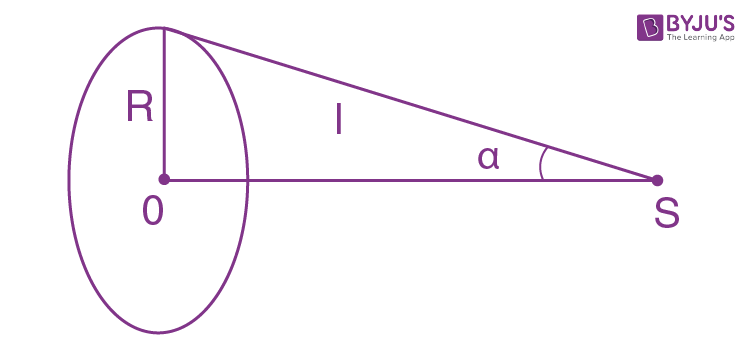The required flux of acoustic power is then Q = Ω/4π

Where Ω is the solid angle subtended by the disc enclosed by the ring at S. This solid angle is

Ω = 2 π(1- cos α)

So flux, ϕ = Io(1- l/ √(r2+R2) 2 πl2

Or ϕ = 1.99 μW.

Let SO be the polar axis. Then the required solid angle is the area of that part of the surface a sphere of much radius whose colatitude is ≤ α.

So, Ω = 2π(1-cos α).

14: A point isotropic source with sonic power P = 0.10 W is located at the centre of a round hollow cylinder with radius R = 1.0 m and height h = 2.0 m. Assuming the sound to be completely absorbed by the walls of the cylinder, find the mean energy flow reaching the lateral surface of the cylinder.

Solution:

1. From the result of power flowing out through anyone of the opening

$=\frac{P}{2}[1 - \frac{h/2}{\sqrt{R^2+(h/2)^2}} ]$

As total power output equals P, so the power reaching the lateral surface must be

= Ph/√[4R2+h2] = 0.07 W

15: The equation of a plane standing wave in a homogeneous elastic medium has the form ξ = a cos kx cos ωt. Plot:

(a) ξ and $\frac{\partial \xi}{\partial x}$ as functions of x at the moments t = 0 and t = T/2, where T is the oscillation period;

(b) the distribution of density ρ(x) of the medium at the moments t = 0 and t = T/2 in the case of longitudinal oscillations;

(c) the velocity distribution of particles of the medium at the moment t = T/4; indicate the directions of velocities at the antinodes, both for longitudinal and transverse oscillations.

Solution:

1. We are given

ξ = a cos kx cos ωt

$\frac{\partial \xi}{\partial x}$ = -a k sin kx cos ωt and

$\frac{\partial \xi}{\partial t}$ = -a ω sin kx sin ωt

Thus, (ξ)t=0 = a cos kx cos ωt and (ξ)t=T/2 = -a cos kx

(partial derivative of ξ with x)t=0 = a k sin kx and (partial derivative of ξ with x)t=T/2 = -a k sin kx

(a) The graph of ξ and $\frac{\partial \xi}{\partial x}$ is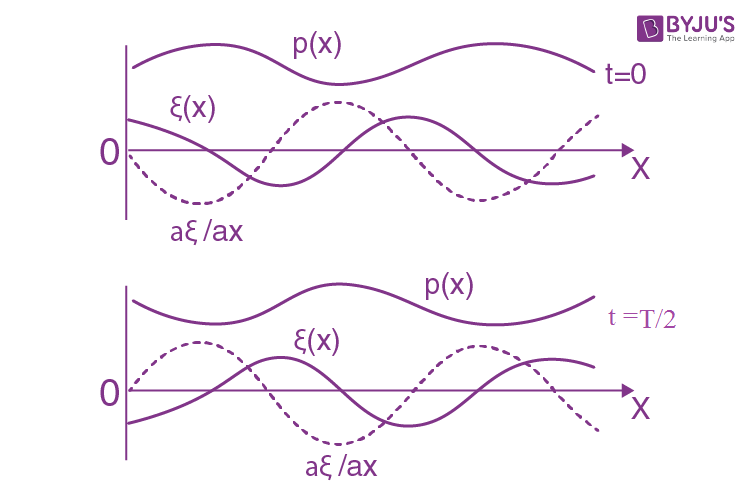(b) We can calculate the density as,

Take a parallelepiped of cross section unity and length dx with its edges at x and x + dx. After the oscillation the edge at x goes to x + ξ (x) and the edge at x + d x goes to

x + dx + ξ(x+dx)

=x + dx + ξ(x) +$\frac{\partial \xi}{\partial x}$ dx

Thus the volume of the element (originally dx) becomes (1 + $\frac{\partial \xi}{\partial x}$)dx

And hence the density becomes ρ = ρo/(1 + $\frac{\partial \xi}{\partial x}$)

On substituting we get for the density p (x) the curves. Referred to above figure.

(c) The velocity v(x) at time t = T/4 is

(1 + $\frac{\partial \xi}{\partial x}$)t=T/4 = -a ω cos kx

On plotting, we get below figure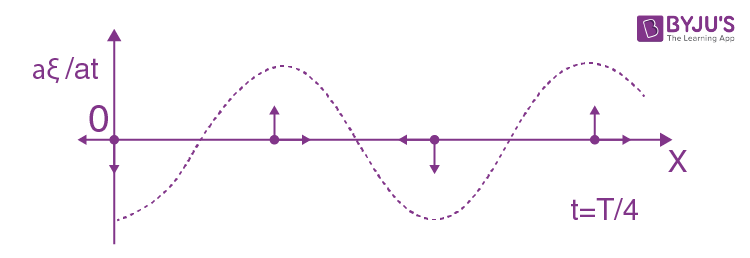16: A longitudinal standing wave ξ = a cos kx- cos ωt is maintained in a homogeneous medium of density ρ. Find the expressions for the space density of

(a) potential energy wp (x, t);

(b) kinetic energy wk (x, t).

Plot the space density distribution of the total energy w in the space between the displacement nodes at the moments t = 0 and t = T/4, where T is the oscillation period.

Solution:

1. ξ = a cos kx- cos ωt

The potential energy density (per unit volume) is the energy of longitudinal strain.

wp= (1/2) stress x strain = (1/2) E($\frac{\partial \xi}{\partial x}$)2,

Here $\frac{\partial \xi}{\partial x}$) is the longitudinal strain

wp= (1/2) Ea2k2 sin2 kx cos2 ωt

But ω2/k2 = E/ρ or Ek2 = ω2ρ

Thus, wp= (1/2) a2 ω2ρ sin2 kx cos2 ωt

(b) The kinetic energy density is

(1/2) ρ ($\frac{\partial \xi}{\partial x}$)2 = (1/2)a2 ω2ρ sin2 kx cos2 ωt

On plotting, we get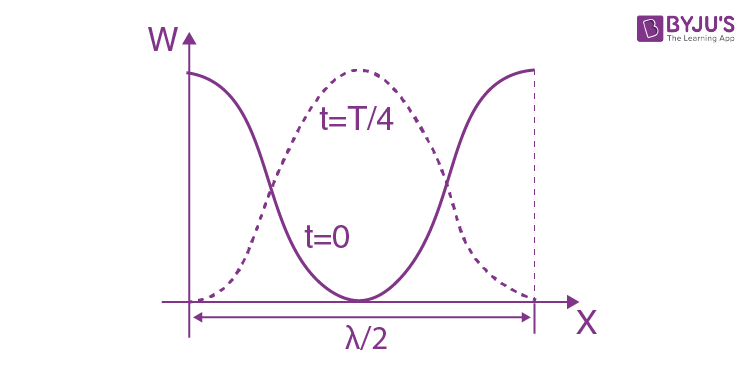For example, at t=o

w=wp+wk = (1/2) a2 ω2ρ sin2 kx

And displacement nodes are at x = π/2k and x =- π/2k.

17: A string 120 cm in length sustains a standing wave, with the points of the string at which the displacement amplitude is equal to 3.5 mm being separated by 15.0 cm. Find the maximum displacement amplitude. To which overtone do these oscillations correspond?

Solution:

1. Let us denote the displacement of the elements of the string by

ξ = s sin kx cosωt

Since the string is 120 cm long we must have k.120 = n π

If x1 is the distance at which the displacement amplitude first equals 3.5 mm then

kx1+15k=π-kx1 or kx1 = (π-15k)/2

One can convince our self that the string has the form shown below: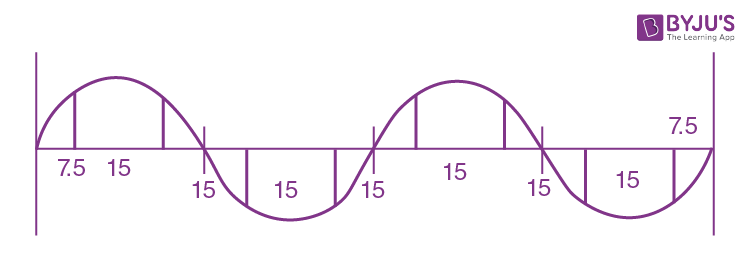It shows that k x 120 = 4π or k = π/30 cm-1

Also, kx1 = π/4 so a = 4.949 mm (approx.)

18: Find the ratio of the fundamental tone frequencies of two identical strings after one of them was stretched by η1 = 2.0% and the other, by η2 = 4.0%. The tension is assumed to 'be proportional to the elongation.

Solution:

1. We have, n = (1/2l)√(T/m) = (1/2l)√(Tl/M)

Here M = total mass of the wire.

When the write is stretched, total mass of the wire remains constant. For the first wire the new length is 1 + η1 and for record wire, the length is l+ η2 l. Also, T1 = α(η, l) where α is constant and T2 = α (η2 l). Substituting in the above formula.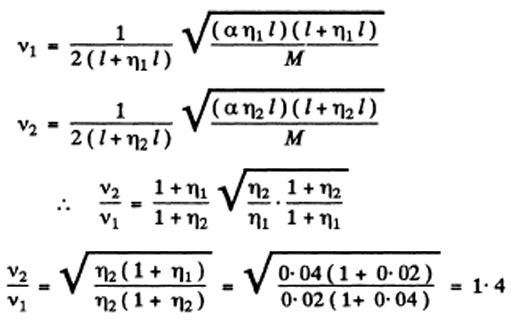19: Determine in what way and how many times will the fundamental tone frequency of a stretched wire change if its length is shortened by 35% and the tension increased by 70%.

Solution:

1. Let initial length and tension be l and T respectively.

v1 = (1/2l)√(T/ρ1)

l' = l - (lx35)/100 = 0.65 l

and new tension, T' = T = (Tx70)/100 = 1.7 T

The new frequency, v2 = (1/2l') √(T’/ρ1) = 1/[2x0.65 l] √(1.7 T/ρ1)

v2/v1 = √(1.7)/0.65 = 2

20: To determine the sound propagation velocity in air by acoustic resonance technique one can use a pipe with a piston and a sonic membrane closing one of its ends. Find the velocity of sound if the distance between the adjacent positions of the piston at which resonance is observed at a frequency v = 2000 Hz is equal to l = 8.5 cm.

Solution:

1. Obviously in this case the velocity of sound propagation

v - 2v (l2 - l1) where l2 and l1 are consecutive lengths at which resonance occur. In our problem, (l2 - l1) = l

v=2vl = 2x2000x8.5 cm/s = 0.34 km/s